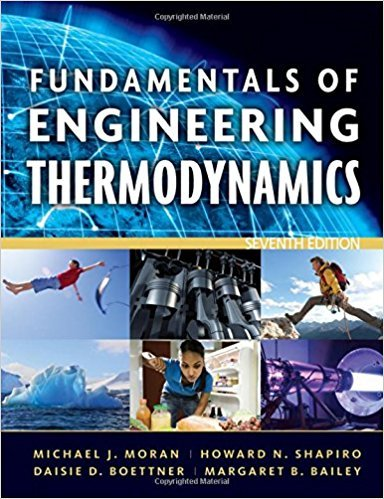×
Get Full Access to Fundamentals Of Engineering Thermodynamics - 7 Edition - Chapter 3 - Problem 54p
Get Full Access to Fundamentals Of Engineering Thermodynamics - 7 Edition - Chapter 3 - Problem 54p

×

# Applying the Energy BalanceWater in a piston-cylinderISBN: 9780470495902 50

## Solution for problem 54P Chapter 3

Fundamentals of Engineering Thermodynamics | 7th Edition

• Textbook Solutions
• 2901 Step-by-step solutions solved by professors and subject experts
• Get 24/7 help from StudySoup virtual teaching assistantsFundamentals of Engineering Thermodynamics | 7th Edition

4 5 1 354 Reviews
22
2
Problem 54P

Problem 54P

Applying the Energy Balance

Water in a piston-cylinder assembly, initially at a temperature of 99.63°C and a quality of 65%, is heated at constant pressure to a temperature of 200°C. If the work during the process is +300 kJ, determine (a) the mass of water, in kg, and (b) the heat transfer, in kJ. Changes in kinetic and potential energy are negligible.

Step-by-Step Solution:
Step 1 of 3

Step 2 of 3

Step 3 of 3

##### ISBN: 9780470495902

Fundamentals of Engineering Thermodynamics was written by and is associated to the ISBN: 9780470495902. This textbook survival guide was created for the textbook: Fundamentals of Engineering Thermodynamics, edition: 7. Since the solution to 54P from 3 chapter was answered, more than 500 students have viewed the full step-by-step answer. The full step-by-step solution to problem: 54P from chapter: 3 was answered by , our top Engineering and Tech solution expert on 07/20/17, 09:01AM. The answer to “Applying the Energy BalanceWater in a piston-cylinder assembly, initially at a temperature of 99.63°C and a quality of 65%, is heated at constant pressure to a temperature of 200°C. If the work during the process is +300 kJ, determine (a) the mass of water, in kg, and (b) the heat transfer, in kJ. Changes in kinetic and potential energy are negligible.” is broken down into a number of easy to follow steps, and 61 words. This full solution covers the following key subjects: Energy, Water, temperature, kinetic, constant. This expansive textbook survival guide covers 14 chapters, and 1501 solutions.

Unlock Textbook Solution